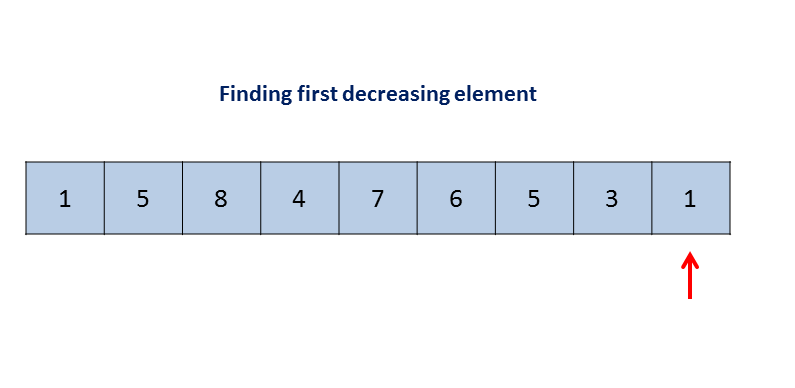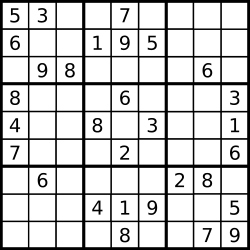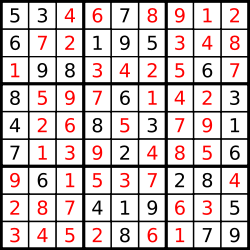# LeetCode(31-40)

## 31. Next Permutation¶

• Array

Implement next permutation, which rearranges numbers into the lexicographically next greater permutation of numbers.

If such arrangement is not possible, it must rearrange it as the lowest possible order (ie, sorted in ascending order).

The replacement must be in-place and use only constant extra memory.

Here are some examples. Inputs are in the left-hand column and its corresponding outputs are in the right-hand column.

1,2,31,3,2
3,2,11,2,3
1,1,51,5,1

Solution

class Solution {
public void nextPermutation(int[] nums) {
int i = nums.length - 2;

while (i >= 0 && (nums[i + 1] <= nums[i])) {
i--;
}

if (i >= 0) {
int j = nums.length - 1;
while (j >= 0 && (nums[j] <= nums[i])) {
j--;
}
swap(nums, i, j);
}
reverse(nums, i + 1);
}

private void swap(int[] nums, int i, int j) {
int k = nums[i];
nums[i] = nums[j];
nums[j] = k;
}

private void reverse(int[] nums, int start) {
int end = nums.length - 1;
while (end > start) {
swap(nums, start, end);
start++;
end--;
}
}
}## 32. Longest Valid Parentheses¶

• String
• Dynamic Programming

Given a string containing just the characters '(' and ')', find the length of the longest valid (well-formed) parentheses substring.

Example 1:

Input: "(()"
Output: 2
Explanation: The longest valid parentheses substring is "()"

Example 2:

Input: ")()())"
Output: 4
Explanation: The longest valid parentheses substring is "()()"

Solution

Approach 1: Using Dynamic Programming

class Solution {
public int longestValidParentheses(String s) {
final int N = s.length();
int[] dp = new int[N];
int longest = 0;

for (int i = 1; i < N; i++) {
if (s.charAt(i) == ')') {
if (s.charAt(i - 1) == '(') {
dp[i] = ((i >= 2) ? dp[i - 2] : 0) + 2;
} else { // s.charAt(i - 1) == ')'
if (i - dp[i - 1] > 0 && s.charAt(i - dp[i - 1] - 1) == '(') {
dp[i] = dp[i - 1] + (i - dp[i - 1] >= 2 ? dp[i - dp[i - 1] - 2] : 0) + 2;
}
}
longest = Math.max(longest, dp[i]);
}
}

return longest;
}
}


1、i-1为'('时，此时我们只需要将i-2的dp值加上'()'的长度2即可。
2、i-1为')'时，dp[i-1]的值就表示了上一个阶段的值，所以i-dp[i-1]就得到了上个阶段起始的坐标。这里我们只要关心i-dp[i-1]-1是'('的情况，因为'('才能和s[i]=')'匹配。因此，dp[i] = dp[i - 1] + dp[i - dp[i - 1] - 2] + 2。

Approach 2: Using Stack

class Solution {
public int longestValidParentheses(String s) {
final int N = s.length();
Stack<Integer> stack = new Stack<>();
int longest = 0;

stack.push(-1);
for (int i = 0; i < N; i++) {
if (s.charAt(i) == '(') {
stack.push(i);
} else {
stack.pop();
if (stack.isEmpty()) {
stack.push(i);
} else {
longest = Math.max(longest, i - stack.peek());
}
}
}

return longest;
}
}


Approach 2.2: Using Stack

class Solution {
public int longestValidParentheses(String s) {
Stack<Integer> stack = new Stack<>();
for (int i = 0; i < s.length(); i++) {
char c = s.charAt(i);
if (c == '(') stack.push(i);
else if (!stack.empty() && s.charAt(stack.peek()) == '(') stack.pop();
else stack.push(i);
}
if (stack.empty()) return s.length();
int res = 0, high = s.length();

while (!stack.empty()) {
int low = stack.pop();
res = Math.max(res, high - low - 1);
high = low;
}
return Math.max(res, high);
}
}


Approach 3: Without extra space

public class Solution {
public int longestValidParentheses(String s) {
int left = 0, right = 0, maxlength = 0;
for (int i = 0; i < s.length(); i++) {
if (s.charAt(i) == '(') {
left++;
} else {
right++;
}
if (left == right) {
maxlength = Math.max(maxlength, 2 * right);
} else if (right >= left) {
left = right = 0;
}
}
left = right = 0;
for (int i = s.length() - 1; i >= 0; i--) {
if (s.charAt(i) == '(') {
left++;
} else {
right++;
}
if (left == right) {
maxlength = Math.max(maxlength, 2 * left);
} else if (left >= right) {
left = right = 0;
}
}
return maxlength;
}
}


## 33. Search in Rotated Sorted Array¶

• Array
• Binary Search

Suppose an array sorted in ascending order is rotated at some pivot unknown to you beforehand.

(i.e., [0,1,2,4,5,6,7] might become [4,5,6,7,0,1,2]).

You are given a target value to search. If found in the array return its index, otherwise return -1.

You may assume no duplicate exists in the array.

Your algorithm's runtime complexity must be in the order of O(log n).

Example 1:

Input: nums = [4,5,6,7,0,1,2], target = 0
Output: 4

Example 2:

Input: nums = [4,5,6,7,0,1,2], target = 3
Output: -1

Solution

class Solution {
public int search(int[] nums, int target) {
final int N = nums.length;
int lo = 0, hi = nums.length - 1;

while (lo < hi) {
int mid = (lo + hi) / 2;
if (nums[mid] > nums[hi])
lo = mid + 1;
else
hi = mid;
}

int pivot = lo;

lo = 0;
hi = nums.length - 1;
while (lo <= hi) {
int mid = (lo + hi) / 2;
int realMid = (mid + pivot) % N;
if (nums[realMid] == target) return realMid;
if (nums[realMid] < target)
lo = mid + 1;
else
hi = mid - 1;
}

return -1;
}
}


## 34. Find First and Last Position of Element in Sorted Array¶

• Array
• Binary Search

Given an array of integers nums sorted in ascending order, find the starting and ending position of a given target value.

Your algorithm's runtime complexity must be in the order of O(log n).

If the target is not found in the array, return [-1, -1].

Example 1:

Input: nums = [5,7,7,8,8,10], target = 8
Output: [3,4]

Example 2:

Input: nums = [5,7,7,8,8,10], target = 6
Output: [-1,-1]

Solution

class Solution {
// returns leftmost (or rightmost) index at which target should be
// inserted in sorted array nums via binary search.
private int extremeInsertionIndex(int[] nums, int target, boolean left) {
int lo = 0;
int hi = nums.length;

while (lo < hi) {
int mid = (lo + hi) / 2;
if (nums[mid] > target || (left && target == nums[mid])) {
hi = mid;
}
else {
lo = mid+1;
}
}

return lo;
}

public int[] searchRange(int[] nums, int target) {
int[] targetRange = {-1, -1};

int leftIdx = extremeInsertionIndex(nums, target, true);

// assert that leftIdx is within the array bounds and that target
// is actually in nums.
if (leftIdx == nums.length || nums[leftIdx] != target) {
return targetRange;
}

targetRange = leftIdx;
targetRange = extremeInsertionIndex(nums, target, false)-1;

return targetRange;
}
}


## 35. Search Insert Position¶

• Array
• Binary Search

Given a sorted array and a target value, return the index if the target is found. If not, return the index where it would be if it were inserted in order.

You may assume no duplicates in the array.

Example 1:

Input: [1,3,5,6], 5
Output: 2

Example 2:

Input: [1,3,5,6], 2
Output: 1

Example 3:

Input: [1,3,5,6], 7
Output: 4

Example 4:

Input: [1,3,5,6], 0
Output: 0

Solution

class Solution {
public int searchInsert(int[] nums, int target) {
int lo = 0, hi = nums.length - 1;

while (lo <= hi) {
int mid = (lo + hi) / 2;
if (nums[mid] == target) {
return mid;
} else if (nums[mid] > target) {
hi = mid - 1;
} else {
lo = mid + 1;
}
}

return lo;
}
}


## 36. Valid Sudoku¶

• Hash Table
• Hash Set]

Determine if a 9x9 Sudoku board is valid. Only the filled cells need to be validated according to the following rules:

1. Each row must contain the digits 1-9 without repetition.
2. Each column must contain the digits 1-9 without repetition.
3. Each of the 9 3x3 sub-boxes of the grid must contain the digits 1-9 without repetition.A partially filled sudoku which is valid.

The Sudoku board could be partially filled, where empty cells are filled with the character '.'.

Example 1:

Input:
[
["5","3",".",".","7",".",".",".","."],
["6",".",".","1","9","5",".",".","."],
[".","9","8",".",".",".",".","6","."],
["8",".",".",".","6",".",".",".","3"],
["4",".",".","8",".","3",".",".","1"],
["7",".",".",".","2",".",".",".","6"],
[".","6",".",".",".",".","2","8","."],
[".",".",".","4","1","9",".",".","5"],
[".",".",".",".","8",".",".","7","9"]
]
Output: true

Example 2:

Input:
[
["8","3",".",".","7",".",".",".","."],
["6",".",".","1","9","5",".",".","."],
[".","9","8",".",".",".",".","6","."],
["8",".",".",".","6",".",".",".","3"],
["4",".",".","8",".","3",".",".","1"],
["7",".",".",".","2",".",".",".","6"],
[".","6",".",".",".",".","2","8","."],
[".",".",".","4","1","9",".",".","5"],
[".",".",".",".","8",".",".","7","9"]
]
Output: false
Explanation: Same as Example 1, except with the 5 in the top left corner being modified to 8. Since there are two 8's in the top left 3x3 sub-box, it is invalid.

Note:

• A Sudoku board (partially filled) could be valid but is not necessarily solvable.
• Only the filled cells need to be validated according to the mentioned rules.
• The given board contain only digits 1-9 and the character '.'.
• The given board size is always 9x9.

Solution

HashTable

class Solution {
public boolean isValidSudoku(char[][] board) {
final int N = 9;
Map<Integer, Character>[] rowMaps = new HashMap[N];
Map<Integer, Character>[] colMaps = new HashMap[N];
for (int i = 0; i < N; i++) {
rowMaps[i] = new HashMap<Integer, Character>(N);
colMaps[i] = new HashMap<Integer, Character>(N);
}

for (int i = 0; i < N; i++) {
for(int j = 0; j < N; j++) {
char ch = board[i][j];
if (ch == '.') {
continue;
} else if (!rowMaps[i].containsValue(ch) && !colMaps[j].containsValue(ch)) {
rowMaps[i].put(j, ch);
colMaps[j].put(i, ch);
} else {
return false;
}
}
}

return checkBox(board, N);
}

private boolean checkBox(char[][] board, int N) {
Map<Integer, Character> map = new HashMap<Integer, Character>(N);
for (int i = 0; i < N; i+= 3) {
for (int j = 0; j < N; j += 3) {
map.clear();
for (int r = i; r < i + 3; r++) {
for (int l = j; l < j + 3; l++) {
char ch = board[r][l];
if (ch == '.') {
continue;
} else if (!map.containsValue(ch)) {
map.put((r - i) * 3 + (l - j), ch);
} else {
return false;
}
}
}
}
}

return true;
}
}


HashSet

class Solution {
public boolean isValidSudoku(char[][] board) {
final int N = 9;
Set<String> set = new HashSet();

for (int i = 0; i < N; i++) {
for (int j = 0; j < N; j++) {
char ch = board[i][j];
if (ch != '.') {
if (!set.add(ch + " in row " + i) ||
!set.add(ch + " in col " + j) ||
!set.add(ch + " in box " + (i / 3) + "-" + (j / 3))) {
return false;
}
}
}
}

return true;
}
}


HashTable的解法比较自然，HashSet的解法更加牛逼。但是runtime差不多。

## 37. Sudoku Solver¶

• Hash Table
• Backtracking

Determine if a 9x9 Sudoku board is valid. Only the filled cells need to be validated according to the following rules:

1. Each row must contain the digits 1-9 without repetition.
2. Each column must contain the digits 1-9 without repetition.
3. Each of the 9 3x3 sub-boxes of the grid must contain the digits 1-9 without repetition.A sudoku puzzle......and its solution numbers marked in red.

Note:

• The given board contain only digits 1-9 and the character '.'.
• You may assume that the given Sudoku puzzle will have a single unique solution.
• The given board size is always 9x9.

Solution

class Solution {
static final int N = 9;

public boolean solveSudoku(char[][] board) {
for (int i = 0; i < N; i++) {
for (int j = 0; j < N; j++) {
char ch = board[i][j];
if (ch != '.') continue;
for (char c = '1'; c <= '9'; c++) {
if (isValid(board, i, j, c)) {
board[i][j] = c;
if (solveSudoku(board)) {
return true;
} else {
board[i][j] = '.';
}
}
}

return false;
}
}

return true;
}

private boolean isValid(char[][] board, int row, int col, char ch) {
for (int i = 0; i < N; i++) {
// check row
if (board[row][i] == ch) return false;
// check col
if (board[i][col] == ch) return false;
// check box
if (board[row / 3 * 3 + i / 3][col / 3 * 3 + i % 3] == ch) return false;
}

return true;
}
}


1、遍历每一个格子，在当前格子没有值的情况下尝试放置1~9
2.1、如果可以放置，在此基础上递归调用求解算法，如果算法返回true则求解成功，否则清除放置的值，继续下次循环
2.2、如果都不能放置，返回false

## 38. Count and Say¶

• String

The count-and-say sequence is the sequence of integers with the first five terms as following:

1.        1
2.        11
3.        21
4.        1211
5.        111221
6.        312211
7.        13112221
8.        1113213211
9.        31131211131221
10.        13211311123113112211

1 is read off as "one 1" or 11.
11 is read off as "two 1s" or 21.
21 is read off as "one 2, then one 1" or 1211.

Given an integer n where 1 ≤ n ≤ 30, generate the *n*th term of the count-and-say sequence.

Note: Each term of the sequence of integers will be represented as a string.

Solution

class Solution {
public String countAndSay(int n) {
String result = "1";

for (int i = 2; i <= n; i++) {
result = countAndSay(result);
}

return result;
}

private String countAndSay(String str) {
final int N = str.length();

int count = 1;
char first = str.charAt(0);
StringBuilder result = new StringBuilder();
for (int i = 1; i <= N; i++) {
if (i != N && str.charAt(i) == first) {
count++;
} else {
result.append(count).append(first);
if (i != N) {
count = 1;
first = str.charAt(i);
}
}
}

return result.toString();
}
}


## 39. Combination Sum¶

• Array
• Backtracking

Given a set of candidate numbers (candidates) (without duplicates) and a target number (target), find all unique combinations in candidates where the candidate numbers sums to target.

The same repeated number may be chosen from candidates unlimited number of times.

Note:

• All numbers (including target) will be positive integers.
• The solution set must not contain duplicate combinations.

Example 1:

Input: candidates = [2,3,6,7], target = 7,
A solution set is:
[
,
[2,2,3]
]

Example 2:

Input: candidates = [2,3,5], target = 8,
A solution set is:
[
[2,2,2,2],
[2,3,3],
[3,5]
]

Solution

class Solution {
public List<List<Integer>> combinationSum(int[] candidates, int target) {
List<List<Integer>> result = new ArrayList<>();
Arrays.sort(candidates);
backtrace(result, new ArrayList<Integer>(), candidates, target, 0);
return result;
}

private void backtrace(List<List<Integer>> result, List<Integer> tmp, int[] candidates, int remain, int start) {
if (remain < 0)
return;
else if (remain == 0)
else
for (int i = start; i < candidates.length; i++) {
backtrace(result, tmp, candidates, remain - candidates[i],  i);
tmp.remove(tmp.size() - 1);
}
}
}


## 40. Combination Sum II¶

• Array
• Backtracking

Given a set of candidate numbers (candidates) and a target number (target), find all unique combinations in candidates where the candidate numbers sums to target.

Each number in candidates may only be used once in the combination.

Note:

• All numbers (including target) will be positive integers.
• The solution set must not contain duplicate combinations.

Example 1:

Input: candidates = [10,1,2,7,6,1,5], target = 8,
A solution set is:
[
[1, 7],
[1, 2, 5],
[2, 6],
[1, 1, 6]
]

Example 2:

Input: candidates = [2,5,2,1,2], target = 5,
A solution set is:
[
[1,2,2],

]

Solution

class Solution {
public List<List<Integer>> combinationSum2(int[] candidates, int target) {
List<List<Integer>> result = new ArrayList<>();
Arrays.sort(candidates);
backtrace(result, new ArrayList<Integer>(), candidates, target, 0);
return result;
}

private void backtrace(List<List<Integer>> result, List<Integer> tmp, int[] candidates, int remain, int start) {
if (remain < 0) {
return;
} else if (remain == 0) {
} else {
for (int i = start; i < candidates.length; i++) {
if(i > start && candidates[i] == candidates[i-1]) continue; // skip duplicates
backtrace(result, tmp, candidates, remain - candidates[i], i + 1);
tmp.remove(tmp.size() - 1);
}
}
}
}


if(i > start && candidates[i] == candidates[i-1])非常容易令人困惑。
[0..start-1]已经处理了，当i>start时，我们已经尝试过了[start, i-1]之间的元素了，此时candidates[i] == candidates[i-1]可以略过。